Download Presentation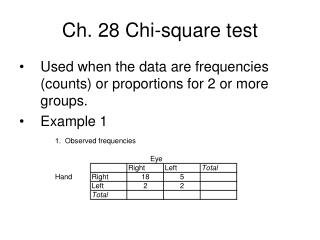Ch. 28 Chi-square test

# Ch. 28 Chi-square test - PowerPoint PPT Presentation

Ch. 28 Chi-square test. Used when the data are frequencies (counts) or proportions for 2 or more groups. Example 1. 5. Calculate ((observed-expected)^2) expected 6. Calculate Chi-square = sum of the values from step 5. The degrees of freedom =I am the owner, or an agent authorized to act on behalf of the owner, of the copyrighted work described.
Download Presentation## Ch. 28 Chi-square test

An Image/Link below is provided (as is) to download presentation

Download Policy: Content on the Website is provided to you AS IS for your information and personal use and may not be sold / licensed / shared on other websites without getting consent from its author.While downloading, if for some reason you are not able to download a presentation, the publisher may have deleted the file from their server.

- - - - - - - - - - - - - - - - - - - - - - - - - - E N D - - - - - - - - - - - - - - - - - - - - - - - - - -
Presentation Transcript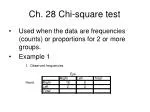Ch. 28 Chi-square test
• Used when the data are frequencies (counts) or proportions for 2 or more groups.
• Example 1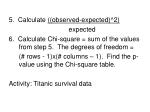5. Calculate ((observed-expected)^2)

expected

6. Calculate Chi-square = sum of the values from step 5. The degrees of freedom =

(# rows - 1)x(# columns – 1). Find the p-value using the Chi-square table.

Activity: Titanic survival data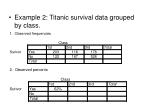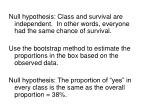Null hypothesis: Class and survival are independent. In other words, everyone had the same chance of survival.

Use the bootstrap method to estimate the proportions in the box based on the observed data.

Null hypothesis: The proportion of “yes” in every class is the same as the overall proportion = 38%.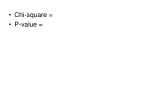Chi-square =
• P-value =